# gvac art .pdf

### File information

This PDF 1.5 document has been generated by TeX / MiKTeX pdfTeX-1.40.13, and has been sent on pdf-archive.com on 14/08/2014 at 23:41, from IP address 79.116.x.x. The current document download page has been viewed 960 times.
File size: 379.23 KB (25 pages).
Privacy: public file

### Document preview

Connection between Gravity and
Electromagnetism
Octavian Balaci
tavib72@mail.com
Abstract
A new interpretation of electrodynamics and gravity is presented,
based on the idea that the electromagnetic and gravitational properties of vacuum are connected. The space and time are treated as
imaginary concepts. With this, the electrodynamics and gravitational
phenomena can be explained with a Galilean invariant vacuum. Also
a new way to explain the gravitational attraction will result.

1

Introduction

The electromagnetic field theory initiated by Faraday and Maxwell presume
that a special medium called luminiferous aether is the bearer of electric and
magnetic fields, aether which was considered at absolute rest in the entire
universe. This model of aether and some variants of it were in contradiction
with many observed phenomena involving the interpretation of electrodynamics in conditions of relative motion. All this problems open the way to
Special Relativity which consider the electromagnetic field at rest relative
to any inertial reference frame. This mean that the electromagnetic field
and the quantum vacuum, which are physical entities, are arbitrary chosen
in regard of their movement stat. But if a physical entity like the quantum
vacuum background is arbitrary relative, then serious problems appear. This
lead to a breaking of causality, an arbitrary change of the coordinate system
will make the vacuum background to behave accordingly without a physical
cause. Also, in this case, other problems exist, like the inability to explain
the aberration of light in the frame of Special or General Relativity. If we
1

take the observer located on earth as frame of reference, the space-time continuum and the electromagnetic field are at rest relative to the observer. As
a consequence, no angle of aberration is predicted in the case of a source
with a spherical emission (star), which is contradicted by well known astronomical observations. The result is different if we analyze the same problem,
considering the star as frame of reference.
A different way to understand electrodynamics and gravity will be presented here, based on an assumed connection between the electromagnetic
and gravitational properties of vacuum. In quantum mechanics the vacuum no longer can be considered pure empty space, but a quantum vacuum
background which is considered Lorentz invariant. However, as we already
point out, Lorentz invariance lead to serious errors. We will show here that
the electrodynamics and gravitational phenomena can be correctly explained
only with a Galilean invariant vacuum background, which not violate causality and will lead us to clear explanations of aberration of light and other
phenomena. The space and time will be considered here as imaginary concepts. The electromagnetic field is immobile in the vacuum background, as
it was in the luminiferous aether and the space-time continuum.

2

Gravitational Vacuum.

The most simple and direct explanation for the deflection of light by gravity,
knowing that the light is an electromagnetic wave, can be done if we assume
that the gravitational potential change the properties of vacuum, which will
produce a gravitational refraction of light. This also will have many other
implications like the change of the speed of light and a new way to explain
the nature of gravitational attraction.
The vacuum is characterized by local properties: gravitational potential
(Γ), electric permittivity (ε0 ), magnetic permeability (µ0 ). In absence of any
ponderable matter, the vacuum properties will be homogeneous distributed
in space, having everywhere the same positive value of gravitational potential, which is the vacuum background gravitational potential. Now if the
ponderable matter, characterized by its heavy mass, is present in space, it
will modify the local gravitational potential of vacuum in accordance with
the Poisson equation of Newtonian gravity
∇2 Γ = 4πGρm
2

(1)

where G gravitational constant, ρm mass density. Assuming a spherical mass
distribution (M ) we can express the gravitational potential at a distance (R)
from the center of mass and outside of it, as follow
Γ = Γ0 −

GM
R

(2)

where Γ0 background gravitational potential in the absence of mass. As we
observe, the gravitational potential of vacuum decrease in the vicinity of
masses and this will modify its electromagnetic properties. The electromagnetic properties of vacuum are not universal constants, but are dependent by
the local gravitational potential. The electric permittivity of vacuum depend
by the gravitational potential as follow
ε0 = κ · f1 (Γ)

(3)

where κ and f1 are undetermined constant and function. The permittivity
also must increase with the proximity to heavy masses, in order to explain
the gravitational refraction of light and the gravitational force. The magnetic
permeability must depend by the gravitational potential in a manner similar
of permittivity. To explain this we consider an electromagnetic wave which
propagate through the vicinity of a heavy cosmic body, the wave direction
is deflected due to the variation of electromagnetic properties (gravitational
refraction), but not suffer reflections, because such a phenomenon has not
been observed. Consequently we can assume that the electromagnetic waves
not suffer reflections when traversing zones with different vacuum properties, which can happen only if the vacuum impedance is independent by the
gravitational potential.
s
µ0
(4)
Z0 =
ε0
µ0 = Z02 ε0 = Z02 κ · f1 (Γ)

(5)

As a result the electric permittivity and the magnetic permeability become
higher and the propagation speed of electromagnetic field become lower in
zones with lower gravitational potential. For determination of constant and
function from (3) we will use the equality between the inertial and heavy
mass, but first we must express the electromagnetic force due to permittivity
variations.

3

2.1

Gravitational Force

One result of spatial variation of electromagnetic properties, is the gravitational force. Let consider a small electric charge characterized only by its
charge q distributed on the surface of a sphere with radius b, at rest, sitting
at a distance R from a heavy mass M. The gravitational potential of vacuum decrease toward the center of mass as described by (2), consequently
the electric permittivity increase. The electric field that surround the charge
(unimportant if positive or negative) contain an amount of energy, if we consider the approximation that the variation of permittivity over the domain
of integration cancel, we have the field energy
Ue0

q2
=
8πε0 b

(6)

The energy of electric field decrease when the charge approach the heavy
mass due to increase of ε0 , the lost energy is converted into work of a force
which is the gravitational attraction force. Because the neutral bodies are
composed from atoms which have internal electric fields, result that they
are attracted too, actually all bodies which have internal electromagnetic
energy experience this attractive force in zones with spatial variable vacuum
properties.
Because the permittivity is no longer equal around the outer area of
sphere, the charged sphere surface no longer have a constant electric potential and tangential components of electric field appear. Supposing that the
electrical charge can move freely on the spherical surface, this charge will
redistribute over the surface until the surface electric potential will be constant, the electric field becoming again normal on surface. Now the charge
density is no longer uniformly distributed over the surface of sphere, being denser where the permittivity is higher and the gravitational potential
lower. Because the electric field is the same over the surface, a net attraction
force appear, fueled by the decreasing electric field energy, representing the
gravitational force.
When the charged sphere move to areas with a lower gravitational potential, the outside oriented electric forces on the surface of sphere will decrease
and the inside oriented forces that hold the charged surface in equilibrium also
must decrease, consequently the gravitational potential must have influence
over the inside forces and over the radius of the charged sphere. In order to
obtain the expected expression for the gravitational force, the charge radius
4

(b) must increase with the decreasing of gravitational potential as follow
b = α · f2 (Γ)

(7)

where α and f2 are the second undetermined constant and function. To
resolve this we consider the charge at rest and make use of the observed
fact that the inertial mass and the heavy mass are equal. Replacing the
permittivity and radius in (6) result
Ue0 =

1
q2
·
8π κα · f1 (Γ) · f2 (Γ)

(8)

The gravitational force must be
Fg0 = −

dUe0
GM
· eR = −mh0 · 2 · eR
dR
R

(9)

where eR is the versor of R, and mh0 is the rest heavy mass of charge q. The
only way to satisfy (9) is
f1 (Γ) · f2 (Γ) =

1
Γ

(10)

with this will result
Fg0 = −

q2

q2
GM
dUe
· eR = −
·
· eR = −
· 2 · eR
dR
8πκα dR
8πκα R

(11)

and the rest heavy mass
q2
(12)
8πκα
At rest the inertial mass depend only by the electric field energy and must
be as follow
Ue0
µ0 q 2
q 2 Z02 κ · f1 (Γ)
mi0 = 2 =
=
·
(13)
c
8πb
8π α · f2 (Γ)
mh0 =

To satisfy the equality mi0 = mh0 , one condition is that the inertial mass
must be independent of the gravitational potential, this lead to f1 (Γ) = f2 (Γ)
and considering (10) result
1
f1 (Γ) = f2 (Γ) = √
Γ
5

(14)

Also we have the equality
q2
q 2 Z02 κ
=
8πα
8πκα

(15)

1
Z0
The inertial and heavy mass at rest, become
κ=

m0 = mi0 = mh0 =

(16)

Z0 q 2
8πα

(17)

Taking into account (16) and (14), we can write the permittivity, the permeability and the speed of light as follow
ε0 =

1

Z0 Γ

Z0
µ0 = √
Γ

c= Γ

(18)
(19)
(20)

From the equation (20) result that the local gravitational potential equal the
square of the local speed of light.
Above was expressed the electrical part of the gravitational force at rest.
At near to rest approximation the magnetic field energy alone have no contribution to the gravitational forces. To account for this we can express the
magnetic field energy of a moving charged sphere relative to the local vacuum
with a low velocity. The magnetic field energy produced by this movement
is
4 Z0 q 2 v 2
4 µ0 q 2 v 2
= ·
(21)
Um0 = ·
3 16πb
3 16πα
which is independent by the gravitational potential. However we used here
near to rest approximation for simplicity, we will show later that, when the
propagation effects are taken into account, the gravitational force, the heavy
and inertial mass are the effect of the total electromagnetic energy.
From the previously description, result that the gravitational force is not
powered by some gravitational field energy, which not exist, but by the body
internal electromagnetic energy. The body internal electromagnetic energy
also contribute to the phenomenon of inertia. This lead to the possibility
that the entire concept of mass may be of electromagnetic nature.
6

2.2

Because the permittivity change with the gravitational potential, this in turn
will lead to the change of atomic radius and the dimensions of bodies. The
radius of an electron orbit around the nucleus is given by the equilibrium
between the nucleus attraction force and the centrifugal inertial force of electron. The quantum effects have an important influence at a such small scale.
Let consider for simplicity the Bohr model of atom, the electron orbital radius
is
4π~2 n2
1
4πε0 ~2 n2
=
· √
(22)
rn =
2
2
Ze me0
Ze me0 Z0 Γ
where Z is the atomic number and Z0 vacuum impedance. The me0 is the
rest mass of electron and is independent by the gravitational potential for low
velocities compared with c. The radius of atom vary with the gravitational
potential in a similar way as the radius of our sphere of charge considered
previously. The atomic radius and dimensions of bodies increase in lower
gravitational potentials.

2.3

The Gravitational Entrainment of Vacuum

One important problem related with the vacuum background is its movement
state. In absence of any ponderable matter, the homogeneous distributed
vacuum background does not have a movement state inside of it and a point
of vacuum background is at rest relative to other point of it. The presence
of ponderable matter will modify the gravitational properties of vacuum and
this properties will follow the movement of ponderable matter. This imply
two possibilities.
The first possibility assume that only the properties are entrained which
require that the variations in the vacuum properties, which follow the ponderable matter position, propagate through it with a finite speed. This will
produce a time lag in the action of gravitational force.
The second possibility assume that the vacuum background behave in
such a way that the gravitational potential of it have no time variations,
which eliminate the propagation and the time lag of the gravitational forces.
This require that the vacuum background must follow the movement of the
gravitational equipotential surfaces. This imply that the entrainment of vacuum is dependent by the local gradient of the gravitational potential. The
material derivative of the gravitational potential at one vacuum point moving
7

with velocity u relative to a mass, is

∂Γ
=
+ u · ∇Γ = 0
Dt
∂t

(23)

The condition of gravitational entrainment request that the material derivative is zero which imply that all variations in the gravitational potential,
including those produced by the partial time derivative, viewed by the vacuum background are zero, the vacuum moving to compensate.
The vacuum entrainment is influenced by the direction and magnitude
of the gravitational acceleration and by the movement of masses, higher the
influence of a heavy body to the gravitational acceleration in one point, lower
the movement of vacuum from that point relative to that body, higher the
entrainment exerted by that body. Below we will analyze two simple cases
of particular interest.
First is the case of a massive body (like a star or planet) far away from
other massive bodies, this body will impose the value of gravitational acceleration in its vicinity, other bodies having only negligible influence because
of their very low masses or very large distances. In this case the vacuum
from the near zone of the massive body (local vacuum), with a negligible
error, is total entrained by this massive body. Small objects with negligible
influence over the gravitational acceleration, moving in the proximity of it,
will experience a “gravitational wind” due to their own movement relative to
the massive body.
The second case is that of rotation of a massive body around an axis of
symmetry. In this case the rotational movement produce no modification
over the gravitational potential in its proximity (if we neglect the centripetal
acceleration), consequently the vacuum will remain unaffected. As a result,
this body itself and any object that rotate with it experience a “gravitational
wind” due to the body rotation around its own axis. However the rotation is
an accelerated motion and because of this the vacuum around the rotating
body will suffer some influences due to this acceleration.
The consequence of vacuum gravitational entrainment over the electromagnetic field, which is immobile relative to vacuum, consist in the advection
of the field by the vacuum movement, leading to the induction of new field
components.

8

3

The Electromagnetic Field and Vacuum

The electric and magnetic fields are immobile relative to the vacuum background, consequently sharing its movement state. The vacuum is used here
as physical entity, relative to which are expressed all velocities which appear
in the electrodynamics equations. We will use the Maxwell-Heaviside equations of electrodynamics, not only because are well known, but also because
are valid in the actual conditions where the permittivity and permeability
are no longer uniformly distributed in space.
The electrical charge q with the volume density ρv may move relative to
vacuum with velocity v, which form the current density j = ρv v. Always a
charge movement through vacuum imply that its electric field will suffer an
advection with velocity -v. Similarly, if a magnet move relative to vacuum
with velocity vm , its magnetic field will suffer an advection with velocity −vm .
A zone of vacuum may move relative to another zone of vacuum (due to
gravitational entrainment) with velocity u, this also produce field advection
between the two zones. Let consider the electric and magnetic field vectors
E,D and H,B, additional medium (like substantial bodies) polarization P and
magnetization M. We have the relations
D = ε0 E + P

(24)

B = µ0 (H + M )

(25)

and
Let consider the spatial elements: volume element dV , surface element dS =
ndS and line element dr, which are tied with the field frame which is the
vacuum. The integral form of electric and magnetic flux equations are

D · dS = q
(26)
and

B · dS = 0

(27)

where q is the charge enclosed inside the surface of integration. Using the
divergence theorem these two equations can be transformed into their local
form
∇ · D = ρv
(28)
9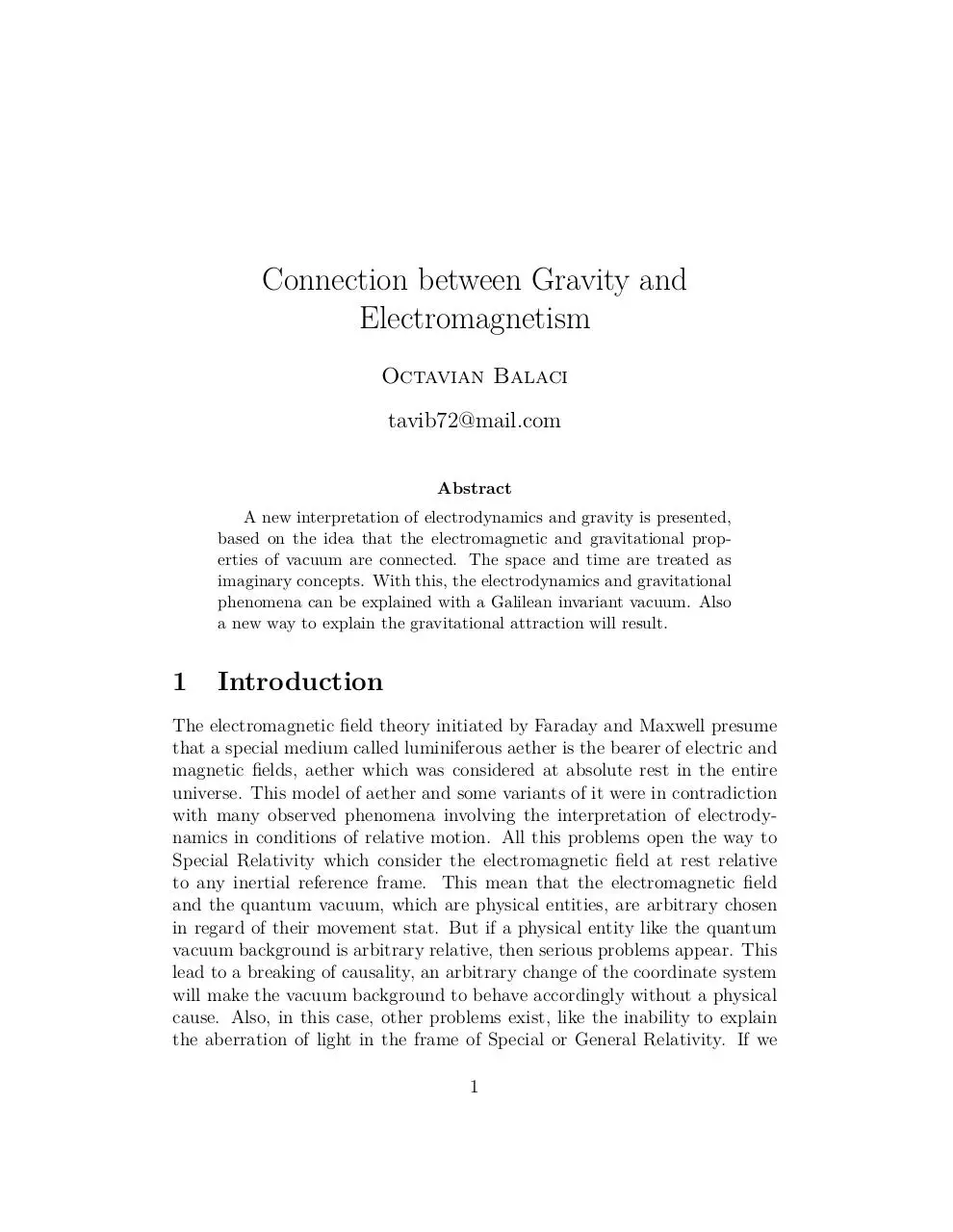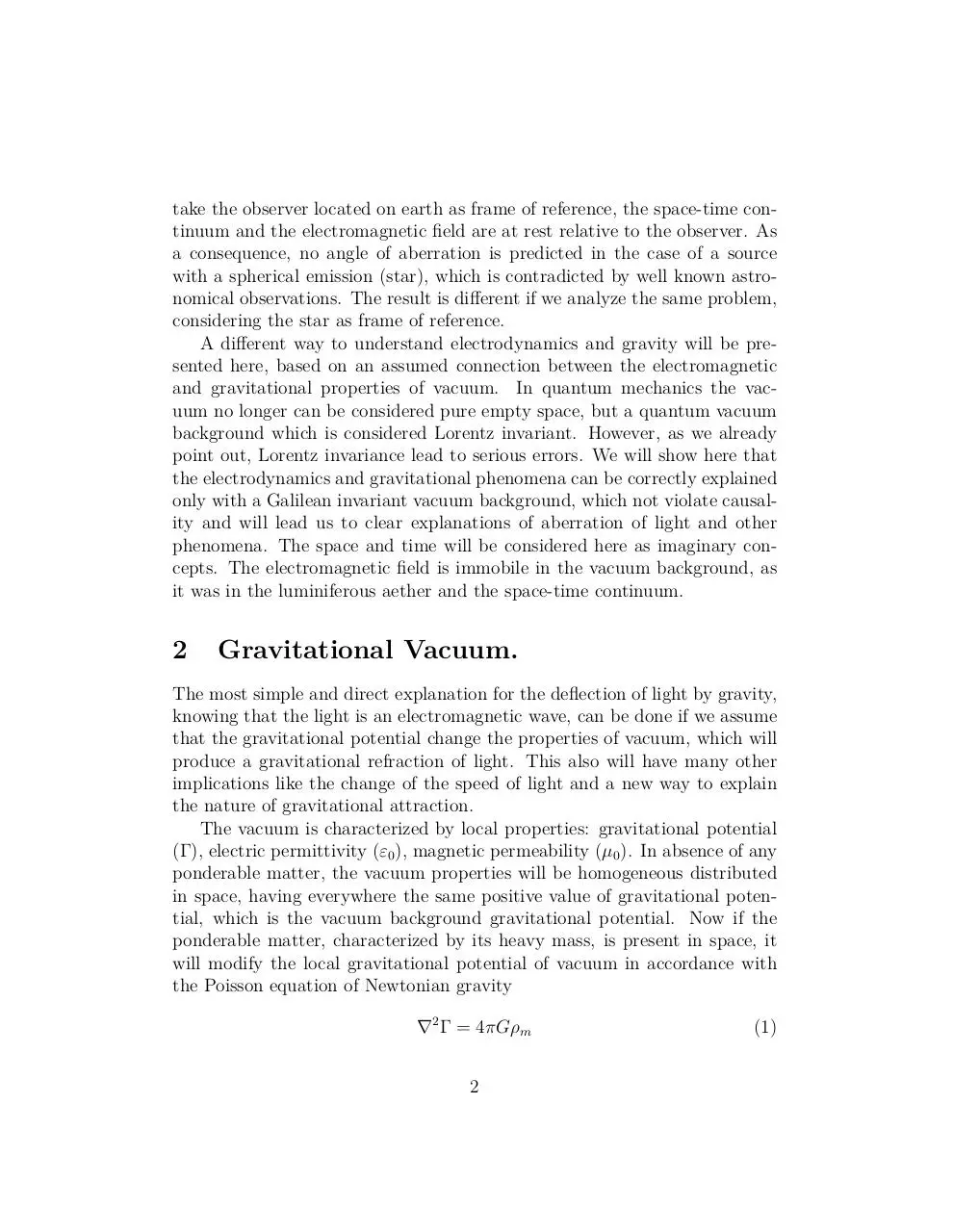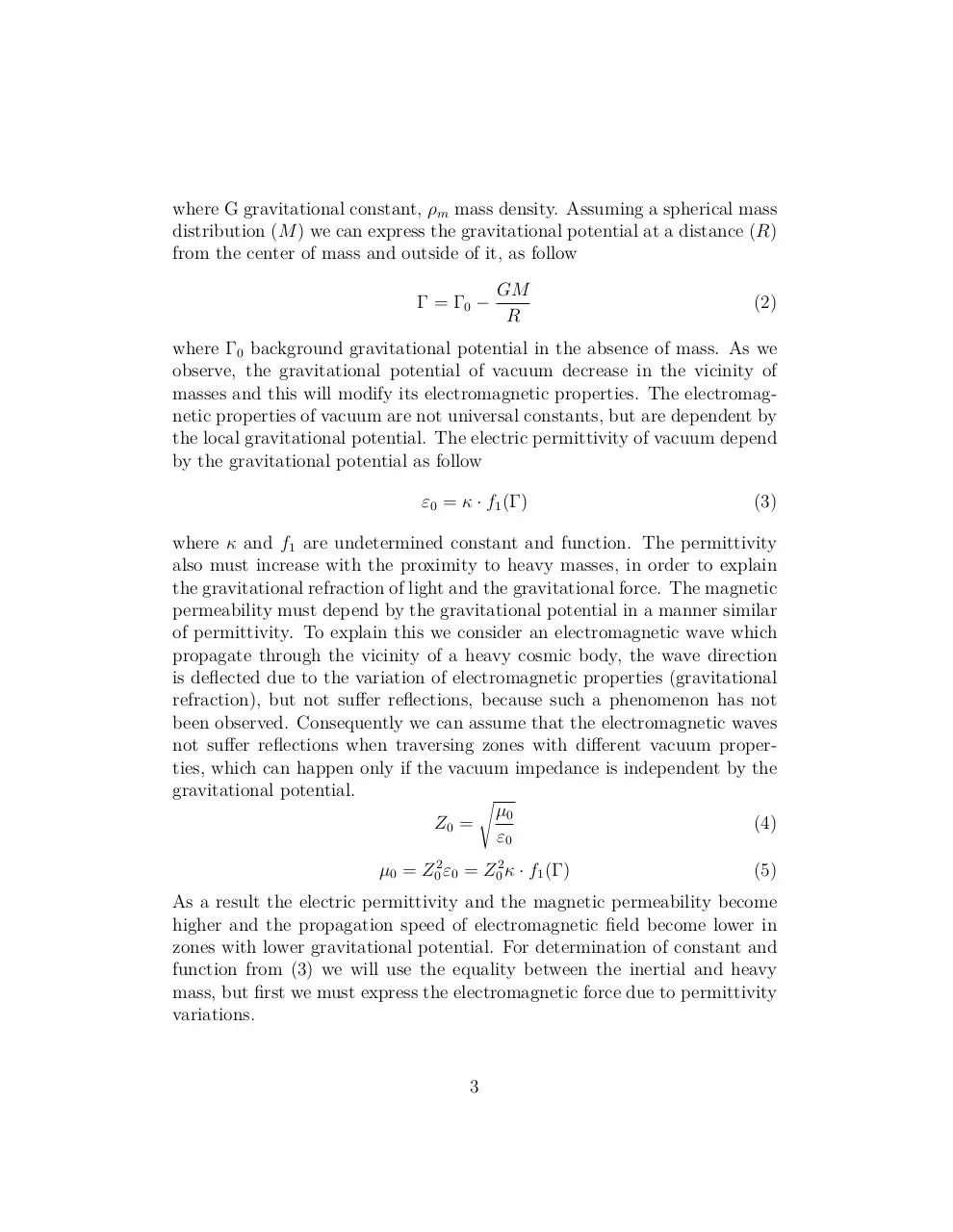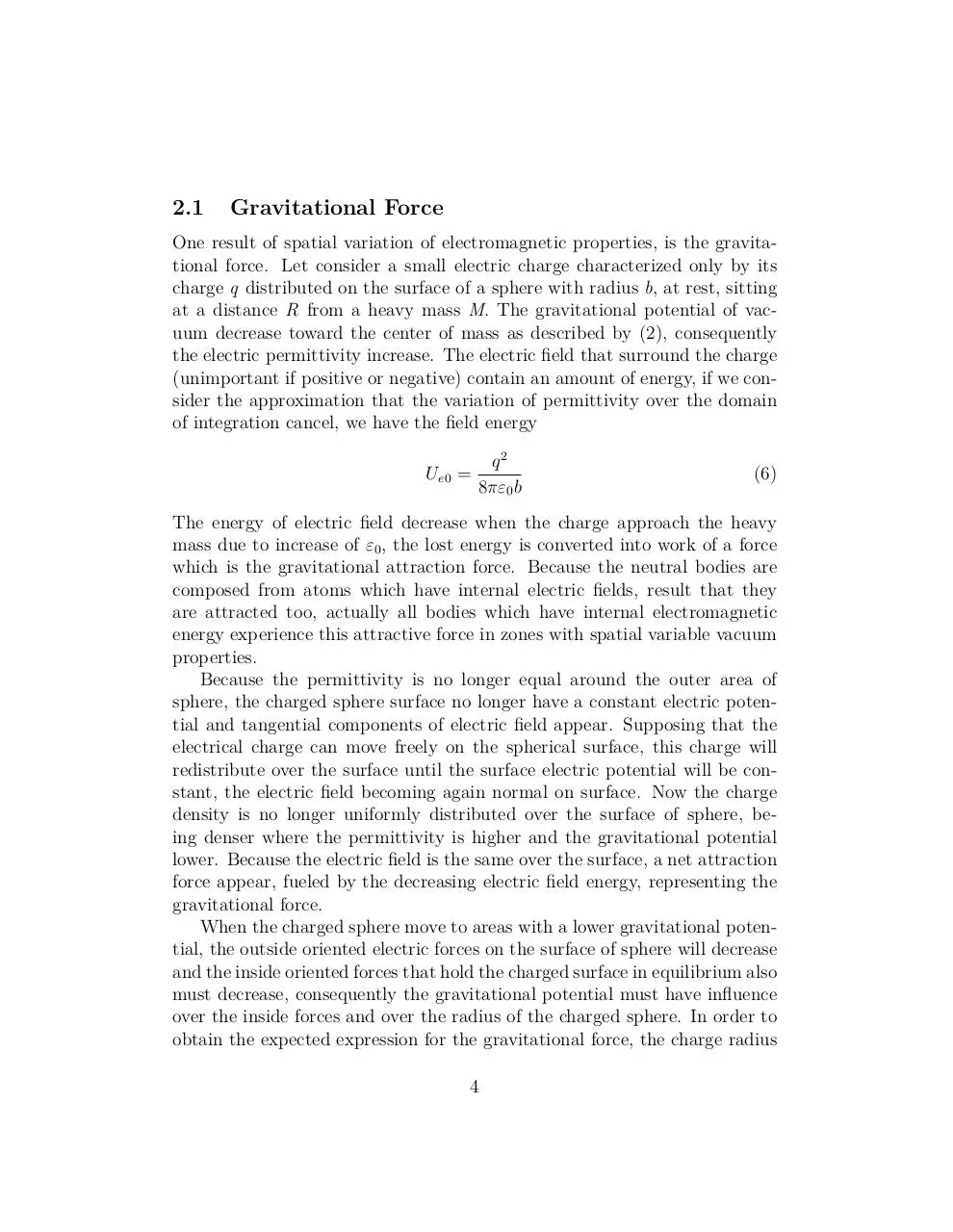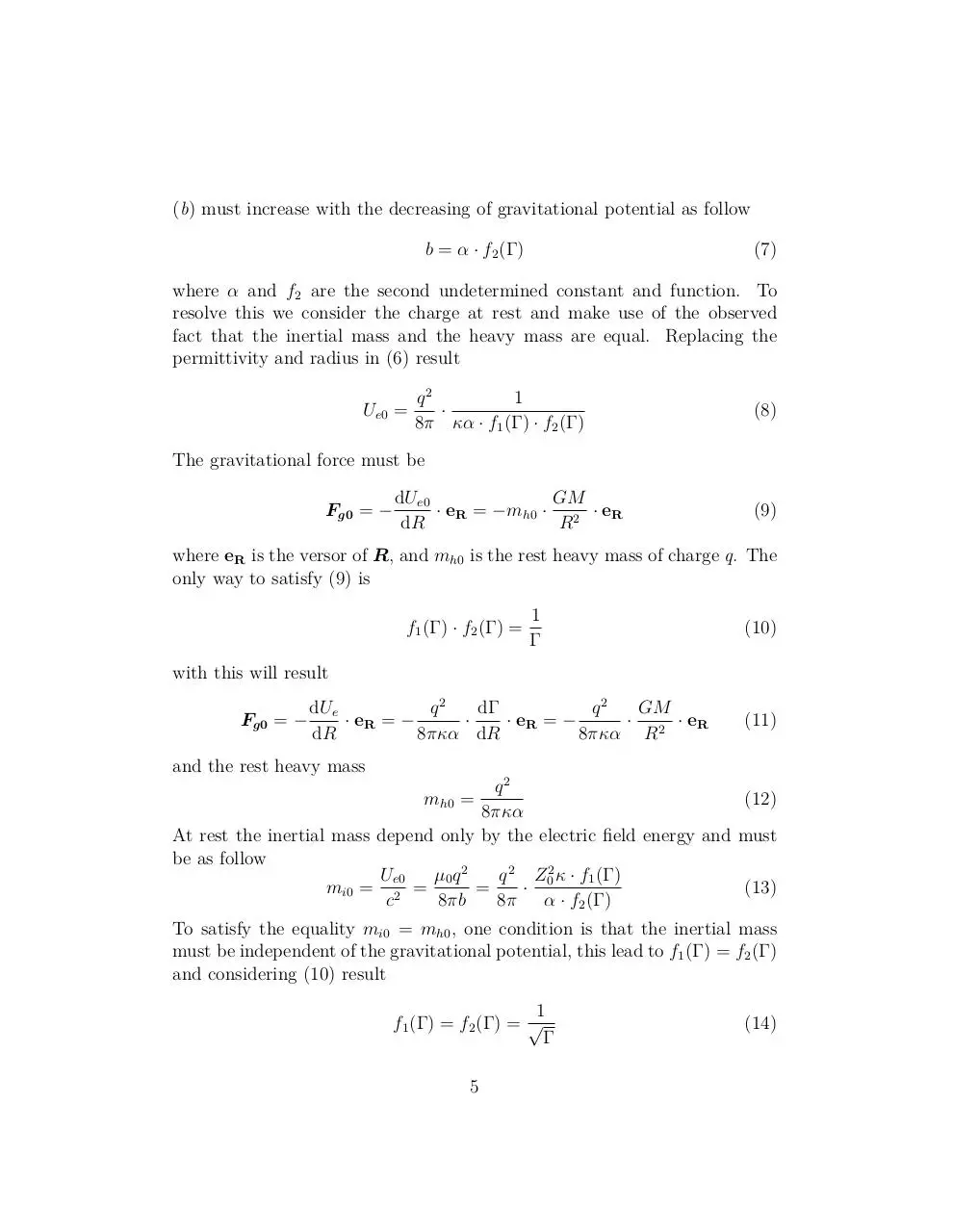gvac-art.pdf (PDF, 379.23 KB)

### Share on social networks

#### HTML Code

Copy the following HTML code to share your document on a Website or Blog